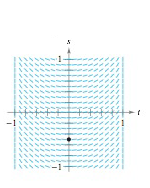Chapter 8.1, Problem 47E

Chapter
Section
Textbook Problem

Slope Field In Exercises 47 and 48, a differential equation, a point, and a slope field are given, (a) Sketch two approximate solutions of the differential equation on the slope field, one of which passes through the given point. (To print an enlarged copy of the graph, go to McthGraphs.com.) (b) Use integration and the given point to find the particular solution of the differential equation and use a graphing utility to graph the solution. Compare the result with the sketch in part (a) that passes through the given point. d s d t = t 1 − x 4 , ( 0 , − 1 2 )(a)

To determine

To calculate: The solution of the differential equation on the slope field.

Explanation

Given:

dsdt=t1t4,(0,12) And the slope field,

Calculation:

Let us consider the differential equation,

dsdt=t1t4

Solve this differential equation for t,

dsdt=t1t4ds=t1t4dt

The above equation is of variable separable form

(b)

To determine

To calculate: The particular solution by finding it.

Still sussing out bartleby?

Check out a sample textbook solution.

See a sample solution

The Solution to Your Study Problems

Bartleby provides explanations to thousands of textbook problems written by our experts, many with advanced degrees!

Get Started

In Exercises 1-6, simplify the expression. (2)3+4(2)212

Calculus: An Applied Approach (MindTap Course List)

Solve the equations in Exercises 126. x44x2=4

Finite Mathematics and Applied Calculus (MindTap Course List)

For the following set of scores, find the value of each expression: a. X b. (X)2 c. X2 d. (X +3)

Essentials of Statistics for The Behavioral Sciences (MindTap Course List)

A population of N = 15 scores has SX = 120. What is the population mean?

Statistics for The Behavioral Sciences (MindTap Course List)

Differentiate. f(z) = (1 ez)(z + ez)

Single Variable Calculus: Early Transcendentals